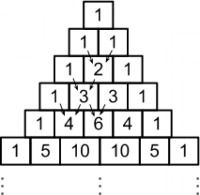{{ message }}

# dcastro / Sequences

A port of Scala's Stream[+A] to C#.

Switch branches/tags
Nothing to show

## Files

Failed to load latest commit information.
Type
Name
Commit time

# Sequences

Sequences is a port of Scala's `Stream[+A]` to C#.

A `Sequence<T>` is an immutable lazy list whose elements are only evaluated when they are needed. A sequence is composed by a head (the first element) and a lazily-evaluated tail (the remaining elements).

The fact that the tail is lazily-evaluated, makes it easy to represent infinite series or sets. For example, here's how to represent the set of all natural numbers.

```public ISequence<int> Naturals(int start)
{
tail: () => Naturals(start + 1));
}

var naturals = Naturals(1);

//take the first 5 natural numbers
naturals.Take(5).ForEach(Console.Write); //prints 12345```

Or, even simpler:

`var naturals = Sequence.From(1);`

Sequences also features memoization, i.e., the sequence stores previously computed values to avoid re-evaluation.

```//start with number 1, and then keep adding 2 to the previous number
var odds = Sequence.Iterate(1, odd =>
{
Console.WriteLine("Adding " + odd + " + 2");
return odd + 2;
});

odds.Take(3).ForEach(Console.WriteLine);
odds.Take(5).ForEach(Console.WriteLine);

//prints
//1
//3
//5

//and then
//1
//3
//5
//7
//9```

You can iterate through an infinite sequence for as long as you want. As long as you don't hold onto its head, each sequence will be elected for garbage collection as soon as you move to the next value. This prevents an infinite sequence from occupying a large and growing ammount of memory.

```foreach (var odd in Sequence.Iterate(1, odd => odd + 2))
{
//when you move to Sequence(11, ?),
//the previous Sequence(9, ?) is elected for collection.
}```

## Examples

The above natural numbers example is very simple. But Sequences allow for so much more. So let's explore some more complex examples.

#### Fibonacci sequence

The Fibonacci sequence is a famous series in mathematics, where each fibonacci number is defined as the sum of the two previous fibonacci numbers, i.e. `F(n) = F(n-1) + F(n-2)`, with seed values `F(0) = 0` and `F(1) = 1`.

In scala, the fibonacci sequence is commonly expressed as follows:

`val fibs: Stream[Int] = 0 #:: 1 #:: fibs.zip(fibs.tail).map { n => n._1 + n._2 }`

In C#, the syntax is a little more verbose, but still readable:

```Func<Tuple<int, int>, int> sum = pair => pair.Item1 + pair.Item2;

ISequence<int> fibs = null;

.Concat(() =>             //and then
fibs.Zip(fibs.Tail)   //zip the sequence with its tail (i.e., (0,1), (1,1), (1,2), (2,3), (3, 5))
.Select(sum));    //select the sum of each pair (i.e., 1, 2, 3, 5, 8)```

The code above creates more objects than needed. The following implementation shows a more efficient way of representing the fibonacci sequence:

```using System.Numerics;

//current and next are any two consecutive fibonacci numbers.
ISequence<BigInteger> Fibs(BigInteger current, BigInteger next)
{
return new Sequence<BigInteger>(current, () => Fibs(next, current + next));
}

var fibs = Fibs(0, 1);

//prints 0 1 1 2 3 5 8 13 21 34
fibs.Take(10).ForEach(Console.WriteLine);```

#### Prime numbers

One way to find every prime number in a given range is to use the Sieve of Eratosthenes. To find the prime numbers up to 100, a slight variation of the sieve goes like this:

2. Let p be the head of the list.
3. Take p as the next prime number, and remove every multiple of p from the list.
4. If the list is empty:
• stop;
• otherwise, repeat from step 2.

Here's a way of implementing the sieve as a sequence.

```var range = Sequence.Range(2, 101);
var primes = PrimesWithin(range);

//prints: 2 3 5 7 11
Console.WriteLine(primes.Take(5).MkString(" "));

public ISequence<int> PrimesWithin(ISequence<int> range)
{
if (range.IsEmpty)
return Sequence.Empty<int>();

//take the next prime number

//skip p, and remove further multiples of p
var filtered = range.Tail.Where(num => num % p != 0).Force();

return new Sequence<int>(p, () => PrimesWithin(filtered));
}```

#### Pascal's Triangle

Everyone knows the famous Pascal's Triangle.The triangle starts with a 1 at the top. In every other row, each number is the sum of the two directly above it.

There are all sorts of ways of representing Pascal's triangle using sequences, but here's an interesting one:

```Func<Tuple<int, int>, int> sum = pair => pair.Item1 + pair.Item2;

Func<ISequence<int>, ISequence<int>> rowFactory =
row => row.Append(0)                //shift row to the left
.Zip(row.Prepend(0))      //shift row to the right, and zip both shifted rows
.Select(sum);             //sum the two shifted rows

var triangle = Sequence.Iterate(
start: Sequence.With(1),
func: rowFactory);```

You start with row (1). From then on, every row is computed by shifting the row to the right, shifting the row to the left, zipping both shifted rows together and producing the sum of each tuple. For example, given the row (1, 3, 3, 1):

``````0 1 3 3 1       //shift right
1 3 3 1 0       //shift left
↓ ↓ ↓ ↓ ↓
1 4 6 4 1
``````

For more examples, refer to the functional tests project.

## Limitations

Due to a limitation in generic type constraints as of C# 5.0, sequences are not covariant, as opposed to Scala's `Stream[+A]`.

Take the signature of `copyToBuffer` in Scala as an example:

`copyToBuffer[B >: A](dest: Buffer[B]): Unit`

The constraint `B >: A` (read A derives from B) cannot be expressed in C# (even though the opposite can be expressed as `where B : A` or B derives from A) unless `A` is also one of copyToBuffer's type parameters - which it isn't.

## Documentation

Documentation is available at dcastro.github.io/Sequences.

## NuGet

To install Sequences, run the following command in the Package Manager Console

``````PM> Install-Package Sequences
``````

A port of Scala's Stream[+A] to C#.

## Releases 2

Release v1.0.1 Latest
May 27, 2014

## Packages 0

No packages published